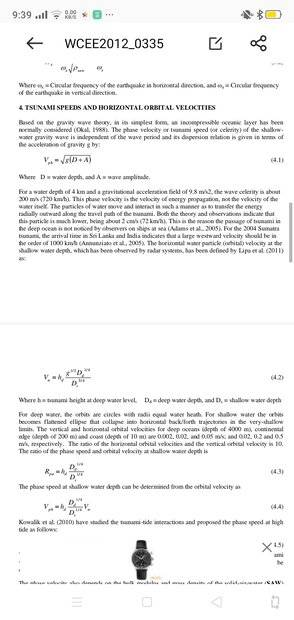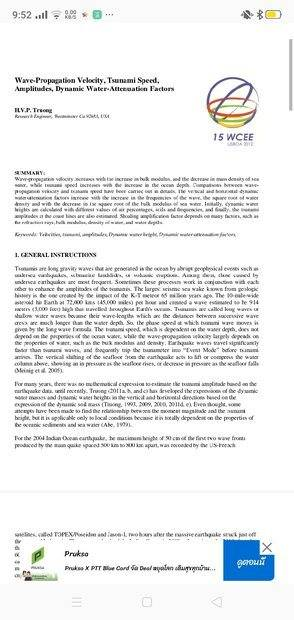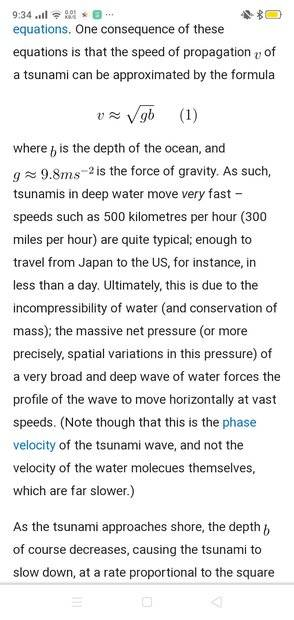# Crest to Crest Velocity of Tsunami

Gold Member
TL;DR Summary
Wavelength for Tsunami is 200 km and velocity is 800 km/hour. What is crest to crest velocity?
A tsunami traveling in deep ocean.
So suppose a tsunami with 200km wavelength is traveling at 800km/hr. Given these are are transverse waves. How is the crest to crest velocity calculated ? For above: 200km/800km/hr would the elapsed time (period) be 15 minutes between passing crests. So there is a crest, then 15 minutes later and 200 km farther there is another crest, correct ?

Last edited:

Homework Helper
Gold Member
That is correct.

Gold Member
Given these are are transverse waves.
This is a popular view of surface water waves ('gravity waves') but in fact there is a great deal of longitudinal displacement as well as vertical displacement. Motion of water particles is in a closed curve - forward and backward and up and down.

Gold Member
Yes and with k = .0314 , w = 25.12, T=.25, wavelength 200km. Then v = wavelength/T = w/k = 800km/hr

Mentor
Sorry for my ignorance, but is a tsunami wave more than just a single impulse? I've never seen a tsunami (arrival) video with more than a single impulse/inundation wave.

Gold Member
Sorry for my ignorance, but is a tsunami wave more than just a single impulse? I've never seen a tsunami (arrival) video with more than a single impulse/inundation wave.
There is one event but it seems able to generate a wave train: Event 1600 km offshore. Wavelength 200km and velocity 800km/hour. T = .25 hr. So in the Two hours the wave is traveling to land wouldn't there be 8 crests/waves in train ? Edit. Maybe one event=one wave

Last edited:
Gold Member
This is a popular view of surface water waves ('gravity waves') but in fact there is a great deal of longitudinal displacement as well as vertical displacement. Motion of water particles is in a closed curve - forward and backward and up and down.
The confusion I have with the tsunami traveling at 800 km/hr(my data above)is that implies that the wave crest is moving across the sea surface at 800km/hr. But this is not the case. In linked paper here author states it is the energy propagation traveling 800km/hr and the crest velocity much lower. See 4Gold Member
From this paperGold Member
@morrobay
Sorry but the resolution on your scan is just not good enough to read. Can you they it with 300dpi please?

•davenn
Gold Member
@morrobay
Sorry but the resolution on your scan is just not good enough to read. Can you they it with 300dpi please?
The paper does not attach. So just search: Wave Propagation, Tsunami Speed... By Truong. Then see 4. Thanks

Gold Member

Gold Member
Gold Member
Thanks.
Which part are you having a problem with? Is it the apparent paradox that the 'slow disturbance' propagates very fast? (as in the sentence
"This phase velocity is the velocity of energy propagation, not the velocity of the water itself.")
Thanks I just woke up 0600. Yes that is the question. So as in post #4 above then the crest velocity does not equal wavelength/T and w/k. Vph = sqrt g(D+A) is required for energy propagation that is independent of wave period.

Last edited:
Gold Member
Thanks I just woke up 0600. Yes that is the question. So as in post #4 above then the crest velocity does not equal wavelength/T and w/k. Vph = sqrt g(D+A) is required for energy propagation that is independent of wave period.
What you are expecting is just not necessary. Imagine a loudspeaker cone that's vibrating at 100Hz. The cone is only moving slowly (peak velocity) if it moves by 5mm - peak speed would be in the order of 0.5m/s. That would be the max mean speed that the air particles would be moving. BUT the wave will leave at 330m/s. So wave speed and particle speed are not the same.

•morrobay
Gold Member
What you are expecting is just not necessary. Imagine a loudspeaker cone that's vibrating at 100Hz. The cone is only moving slowly (peak velocity) if it moves by 5mm - peak speed would be in the order of 0.5m/s. That would be the max mean speed that the air particles would be moving. BUT the wave will leave at 330m/s. So wave speed and particle speed are not the same.
Yes I understood vertical particle displacement in transverse wave propagation. The problem was in attempting to apply v = w/k , radians/sec / radians/m = meters/second to a tsunami wave. Because in other transverse waves w/k equals the phase velocity/ crest velocity. Obviously that would be impossible with a crest velocity 800km/hour on the open seas.
This screenshot explains tsunami propagation.Gold Member
Yes I understood vertical particle displacement in transverse wave propagation.
My comment was about a longitudinal wave. (?)

Gold Member
So wave speed and particle speed are not the same.
Yes I know , I was replying to this last sentence that also applies to transverse waves

•